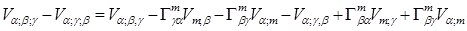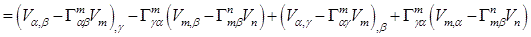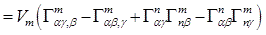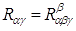## Pages

### 12. Riemann Curvature and Ricci Tensor

This is the final section of the mathematical section part of this report. Having some concept of the basics of the curvilinear system, we are now in position to proceed with the concept of the Riemann Tensor and the Ricci Tensor.

We first start off with the Riemann Tensor. Let us consider a following figure,
In the figure above we can parallel transport the vector $\vec{V}$ two ways around the loop from $A$ to $D$. Now the question we could ask ourselves is that if the vector being transported match up. In the general we would say that the vectors do match up and we wouldn’t doubt our conscience. But this in general wouldn’t be correct, since we are so much familiar to using the Cartesian system of the coordinate. In general there could be various different geometries beside the usual Euclid’s geometry. In the various other geometries, the measure of the difference in the vector is indeed the measure of the deviation from the usual Cartesian coordinate or similarly known as the Riemann curvature tensor of the system.
If we look at the above diagram, in general the mathematical statement equivalent is as follows,

Notice that the semi-colon sign in the equation is the covariant differentiation.We now solve for the above statement and come up with the notion of the curvature tensor.After the partial differential and substituting $V_{n}$ to $V_{m}$ i.e. substituting the dummy index $n$ to $m$, we have the following,Here, $R_{\alpha \beta \gamma }^{m}$ is called the curvature tensor or the Riemann Curvature Tensor and is equal to,

RICCI TENSOR
Now that we have established the Riemann tensor as the notion of the curvature, we may want to relate it to the stress-energy tensor. Unfortunately a $4^{th}$ rank tensor cannot equal a $2^{nd}$ rank one, so we are motivated to consider $2^{nd}$ rank tensor built from Riemann curvature tensor.
If we look expand the curvature tensor, it has a forbidding $256$ components. Luckily several symmetries reduce these substantially. These symmetries reduce the number of independent component to $20$. And these symmetries also mean that there is only one independent contraction to reduce to the second rank tensor or the Ricci tensor.Notice here the indices being contracted; contracting the other indices gives us zero. We can thus write the expression for the Ricci tensor as follows,

If we further contract the Ricci tensor we have the quantity the Ricci scalar.

We can ask ourselves the question what if the Ricci tensor is zero?

The definite answer would be that it implies the space is flat. That is to say that there exists a transformation property between the curvilinear system concerned and the Cartesian system.

Extra note here..I hope this is helpful..